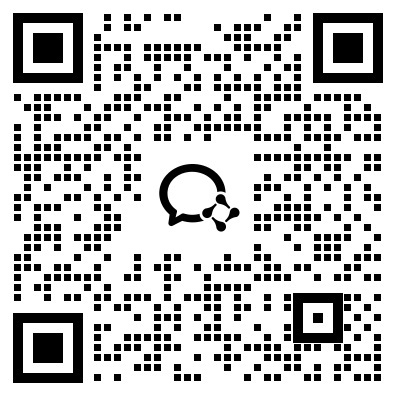﻿ 中山市2019年成人高考高起点数学(文)考试真题及答案_中山成考网

# 中山市2019年成人高考高起点数学(文)考试真题及答案

2019年成人高考高起点数学(文)考试真题及答案

1.设全集U=(1,2,3,4},集合M=(3.4],则CJM =

A.{2,3}B.[2,4}C{L4}D.(1.2}

2.函数y = cos4x的最小正周期为

A.π/4

B.π/2

C.π

D.π

3.设甲: b=0;乙:函数y= kx + b的图像经过坐标原点，则

A.甲是乙的充分条件但不是必要条件

B.甲是乙的必要条件但不是充分条件

C.甲是乙的充要条件

D.甲既不是乙的充分条件也不是乙的必要条件

5.函数y= 根号1- x石的定义域是

A. {x|x≥-1}

B. {x|x≤ 1}

C. {x|x≤-1}

D. {xI-1≤x≤1}

6.设0

A.1<2*x<2

B. 0<2*< 1

C. log1/2x< 0

D. log:x > 0

7.不等式|x+1/2|>1/2的解集为

A. {x|-1

B. {x|x>0或x<-1}

C. {x|x>-1}

D. {x|x< 0}

8.甲、乙、丙、可4人排成一行，其中甲、乙必须排在两端，则不同的排法共有

A.2种

B.4种

C.8种

D.24种

9.若向量a=(1,1), b=(1,-1)，则≌a-gb=A. (-1,2) B. (1,-2)C. (1,2)

D. (-1,-2)

10.log21 + 161/2+(-2)°=

A.5

B.4

C.3

D.2

11.函数y=x≥- 4x- 5的图像与x轴交于A、B两点，则|AB|=

A.3B.4C.5D.6

12.下列函数为奇函數的是A. y=-2x+3B. y=-EC. y=x2-3D. y= 3cosx

13.双曲线”-些= 1的焦点坐标为

A. (-5,0)，。 (5,0)

B. (-V70)，(V70)

C. (0,-5)，60,5)

D. (0，一7)，(0,V7)﻿

## 中山成考网在线客服